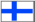Level 2 (upper level)# Theory of modelling

### Bayesian probability theory

In Bayesian statistics, probabilities are interpreted as degrees of belief. The interpretation is wider than in the so called frequentist school, where probabilities are understood as limiting frequencies of events. The wider interpretation makes it possible to describe learning and intelligence with an exact, mathematical language.

#### Basic rules

The basic rules of Bayesian probability theory are the sum and product rule:
P(A | C) + P(¬A | C) = 1
P(AB | C) = P(A | C) P(B | AC)
C means here all the background assumptions. Often C is not denoted:
P(A) + P(¬A) = 1
P(AB) = P(B) P(A | B)

If B1, ..., Bn are n mutually exclusive and exhaustive explanations for A, the marginalisation principle can be derived from the sum and product rule
P(A) = P(AB1) + ... + P(ABn) = P(A | B1) P(B1) + ... + P(A | Bn) P(Bn).
In other words, the probability of A is obtained by going through all the possible explanations for A.

The Bayes' rule tells how the probabilities of hypotheses (explanations) change when A is measured.
P(Bi | A) = P(Bi) P(A | Bi) / P(A)
The probabilities of the explanations which agree well with the observation A are increased.

### Connection with logic

The classical deductive logic deals with inference from rules using binary yes/no truth values. The Bayesian probability theory can be derived from axioms which describe inference with uncertain truth values. The Bayesian probability can thus be seen as an extension of classical logic to uncertain truth values.

This extension also makes possible the description of inductive logic: a set of hypotheses about possible worlds is chosen as premisses and the observations then support some hypotheses and are against some others. Inductiveness is thus embedded in the premisses and the actual inference is deductive. A logic capable of inductive inference must be able to represent uncertainty since usually the observations do not assertain or reject any hypothesis completely but only support or are against to some extent.

### Probabilistic models

Probabilistic models are tool with which it is possible to define a large set of hypotheses about possible worlds that have created the observations. Each model defines a probability distribution p(D | MwI) for the data D (observations). M is the structure of the model and w its parameters. Here the premisses are denoted by I. The posterior distribution P(Mw | DI) of the models and parameters is obtained from Bayes' rule.
p(Mw | DI) = p(Mw | I) p(D | MwI) / p(D | I)
It can be used for predicting new things using marginalisation principle. Often only one structure is selected with neural networks, but, according to Bayesian probability theory, the right way is to use all structures and parameters weighed by the posterior density.

### Approximation of the posterior

If there are a large amount of parameters - as there often is in neural networks - it is usually impossible to represent the posterior density exactly in a useful form. In practice the posterior density has to be approximated. The Gaussian approximation is computationally tractable and it can be shown that the posterior density approaches asymptotically Gaussian distribution as the number of samples grows. If there are very many parameters, it is impractical to represent the full covariance matrix. Assume we are using diagonal covariance. Then we only need to represent the means and variances of the parameters. Let us denote the approximation by q(Mw; imw), where i is the index of the model structure (the approximation thus has non-zero probabilities only for one structure), m the vector of means and v the vector of variances of the parameters.

### Kullback-Leibler information

A measure of the quality of the approximation is needed for selecting the best approximation. Kullback-Leibler information is a measure of the difference between two distributions, hence it is suited for the job. The goal of the learning is to find an approximation q(Mw; imw) for the posterior density p(Mw | DI) which minimises the misfit between q and p, i.e., the Kullback-Leibler information between q and p.

### Connection with information theory

The more complex a model, the better it can represent the data. Still it is not reasonable to choose too complicated models. Assume, for instance, that we have observed the data D. An extreme example of a complicated model is the one that says that the obserevation is D. It explains the observation completely but cannot be used for anything.

Intuitively it is clear that a more simple explanation is better than a more complex if both are equally good in explaining the observations. Information theoretically motivated method Minimum Message Length (MML) expresses this exactly. It deals with the length of description of the data when using a model. First the model is described and then the discrepancy between the model and the data. Their total descpription length is then minimised.

The minimisation of Kullback-Leibler information yields, as a special case, practically the same formula as MML.

Level 2 (upper level)

Updated 13.10.1998
Harri Lappalainen

<Harri.Lappalainen@hut.fi>